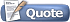Thread: Sampling .wav files View Single Post09-18-2014, 01:05 PM #15 Alkamist Human being with feelings   Join Date: Dec 2011 Posts: 500I think I am close to being able to play a 16 bit wave file at 44.1 kHz. I can build my plugin fine, but when I put it in my plugin folder and try to scan it into Reaper, I get an error message telling me that my plugin executed an invalid operation. Not sure where it is coming from but I am fairly certain it's coming from the sampler portion. The problem came up when I added the LoadWaveFile and BufferSample functions to my constructor. Does anyone have any ideas what might be going wrong? Sampler.h Code: ```#ifndef __SAMPLER__ #define __SAMPLER__ #include "GallantSignal.h" using Gallant::Signal0; #include #include #include class Sampler { public: void LoadWaveFile(char *fname); void BufferSample(); void NextSample(double &leftOutput, double &rightOutput); void Reset(); Signal0<> FinishedPlayingSample; Sampler(void) { LoadWaveFile("c:/Users/Corey/Desktop/Test.wav"); BufferSample(); mPlayhead = 0; } private: int mPlayhead; char *mChunkID, *mChunkSize, *mFormat, *mSubChunkOneID, *mSubChunkOneSize, *mAudioFormat, *mNumChannels, *mSampleRate, *mByteRate, *mBlockAlign, *mBitsPerSample, *mSubChunkTwoID, *mSubChunkTwoSize, *mData; std::ifstream myfile; std::vector mOutputL; std::vector mOutputR; }; #endif /* defined(__SAMPLER__) */``` Sampler.cpp Code: ```#include "Sampler.h" using namespace std; void Sampler::LoadWaveFile(char *fname) { myfile.open(fname, ios::in | ios::binary); if (myfile.is_open()) { mChunkID = new char; myfile.read(mChunkID, 4); //Should have 'RIFF' if (!strcmp(mChunkID, "RIFF")) { //We had 'RIFF', so let's continue mChunkSize = new char; myfile.read(mChunkSize, 4); //Size of the rest of the chunk following this number mFormat = new char; myfile.read(mFormat, 4); //Should contain 'WAVE' if (!strcmp(mFormat,"WAVE")) { //This is probably a wave file since it contained "WAVE" mSubChunkOneID = new char; myfile.read(mSubChunkOneID, 4); //Should contain 'fmt ' mSubChunkOneSize = new char; myfile.read(mSubChunkOneSize, 4); //Size of the rest of the Subchunk following this number mAudioFormat = new char; myfile.read(mAudioFormat, 2); //PCM = 1, other values mean there is some type of file compression mNumChannels = new char; myfile.read(mNumChannels, 2); //1 mono, 2 stereo, etc. mSampleRate = new char; myfile.read(mSampleRate, 4); //The sample rate of the audio mByteRate = new char; myfile.read(mByteRate, 4); //mSampleRate * mNumChannels * mBitsPerSample/8 mBlockAlign = new char; myfile.read(mBlockAlign, 2); //mNumChannels * mBitsPerSample/8 mBitsPerSample = new char; myfile.read(mBitsPerSample, 2); //8 bits = 8, 16 bits = 16, 24 bits = 24, etc. mSubChunkTwoID = new char; myfile.read(mSubChunkTwoID, 4); //Should contain 'data' mSubChunkTwoSize = new char; myfile.read(mSubChunkTwoSize, 4); //How many bytes of sound data we have: //mNumSamples * mNumChannels * mBitsPerSample/8 myfile.read(mData, *mSubChunkTwoSize); //Read in all of the sound data myfile.close(); //Close the file } else cerr << "Error: RIFF file but not a wave file" << endl; } else cerr << "Error: not a RIFF file" << endl; } else cerr << "Error: Could not open the file!" << endl; } void Sampler::BufferSample() { //Make the vectors the same size as the audio data for each channel mOutputL.resize(*mSubChunkTwoSize/2); mOutputR.resize(*mSubChunkTwoSize/2); short int *dataPT; dataPT = (short int *)mData; for (int i = 0, j = 0; i < *mSubChunkTwoSize, j < *mSubChunkTwoSize/2; i += 2, j++) { //The if else statements are to deal with the fact that a short int //has the range of -32768 to 32767. Not sure if this is how I should //do it though. if (dataPT[i] >= 0) { mOutputL[j] = dataPT[i]/32767; } else { mOutputL[j] = dataPT[i]/32768; } if (dataPT[i+1] >= 0) { mOutputR[j] = dataPT[i+1]/32767; } else { mOutputR[j] = dataPT[i+1]/32768; } } } void Sampler::NextSample(double &leftOutput, double &rightOutput) { //The playhead scrolls through the file until it reaches the end. //A signal is then sent that the sample is done and it can free //a voice. leftOutput = mOutputL[mPlayhead]; rightOutput = mOutputR[mPlayhead]; if (mPlayhead == mOutputL.size()) { FinishedPlayingSample(); return; } mPlayhead++; } void Sampler::Reset() { mPlayhead = 0; }```